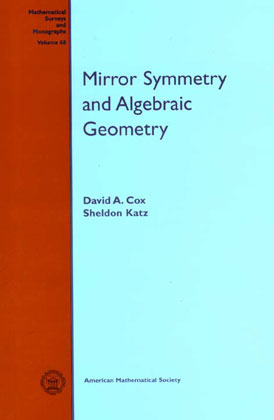#Mirror Symmetry and Algebraic Geometry Mathematical Surveys and Monographs Volume 68 American Mathematical Society, 1999

## What's in the Book

This monograph is an introduction to the mathematics of mirror symmetry, with a special emphasis on its algebro-geometric aspects. Topics covered include the quintic threefold, toric geometry, Hodge theory, complex and Kähler moduli, Gromov-Witten invariants, quantum cohomology, localization in equivariant cohomology, and the recent work of Lian-Liu-Yau and Givental on the Mirror Theorem. The book is written for algebraic geometers and graduate students who want to learn about mirror symmetry. It is also a reference for specialists in the field and background reading for physicists who want to see the mathematical underpinnings of the subject.

## Typographical Errors

Click here for a list of typographical errors in Mirror Symmetry and Algebraic Geometry:

Also, Proposition 5.5.4 on page 98 is incorrect and the proof given on pages 99 and 100 has some gaps. The above list of typographical errors gives the correct statement of the proposition but is not able to fix all of the errors in the proof (the correct proof is a much longer argument which doesn't fit into the space available on page 99). If you want to see the full details of the proof, click here for a postscript file of the proof.

## Contacting the American Mathematical Society

To find Mirror Symmetry and Algebraic Geometry in the AMS on-line catalog, click here .

## Contacting the Authors

You can contact the authors at the following email addresses:

dacox@amherst.edu
katz@math.uiuc.edu